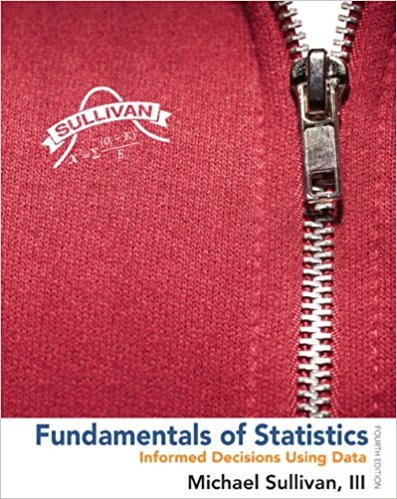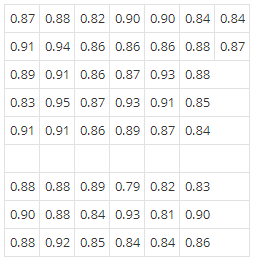×
Get Full Access to Fundamentals Of Statistics - 4 Edition - Chapter 3.2 - Problem 23ayu
Get Full Access to Fundamentals Of Statistics - 4 Edition - Chapter 3.2 - Problem 23ayu

×

# Solved: The Empirical Rule The following data representISBN: 9780321838704 30

## Solution for problem 23AYU Chapter 3.2

Fundamentals of Statistics | 4th Edition

• Textbook Solutions
• 2901 Step-by-step solutions solved by professors and subject experts
• Get 24/7 help from StudySoup virtual teaching assistantsFundamentals of Statistics | 4th Edition

4 5 1 366 Reviews
12
5
Problem 23AYU

The Empirical Rule The following data represent the weights (in grams) of a random sample of 50 M&M plain candies.(a) Determine the sample standard deviation weight. Express your answer rounded to three decimal places.

(b) On the basis of the histogram drawn in Section 3.1, Problem 27, comment on the appropriateness of using the Empirical Rule to make any general statements about the weights of M&Ms.

(c) Use the Empirical Rule to determine the percentage of M&Ms with weights between 0.803 and 0.947 gram.

(d) Determine the actual percentage of M&Ms that weigh between 0.803 and 0.947 gram, inclusive.

(e) Use the Empirical Rule to determine the percentage of M&Ms with weights more than 0.911 gram.

(f) Determine the actual percentage of M&Ms that weigh more than 0.911 gram.

Step-by-Step Solution:

Step 1 of 2

a)

First we need to find mean.=== 0.766

Here standard deviation is== 0.0012

Then the sample variance is

s =s =s = 0.036

Hence the sample variance is s = 0.036 gram.

Step 2 of 2

## Discover and learn what students are asking

Calculus: Early Transcendental Functions : Hyperbolic Functions
?In Exercises 7-14, verify the identity. $$\tanh ^{2} x+\operatorname{sech}^{2} x=1$$

Chemistry: The Central Science : Atoms, Molecules, and Ions
?Are these two compounds isomers? Explain. [Section 2.9]

Statistics: Informed Decisions Using Data : Comparing Three or More Means (One-Way Analysis of Variance)
?Soybean Yield The data in the table represent the number of pods on a random sample of soybean plants for various plot types. An agricultural research

Unlock Textbook Solution